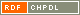# Show document

Title: A complete classification of cubic symmetric graphs of girth 6 Kutnar, Klavdija (Author)Marušič, Dragan (Author)http://dx.doi.org/10.1016/j.jctb.2008.06.001 English Not categorized 1.01 - Original Scientific Article FHŠ - Faculty of Humanities A complete classification of cubic symmetric graphs of girth 6 is given. It is shown that with the exception of the Heawood graph, the Moebius-Kantor graph, the Pappus graph, and the Desargues graph, a cubic symmetric graph ▫$X$▫ of girth 6 is a normal Cayley graph of a generalized dihedral group; in particular, (i) ▫$X$▫ is 2-regular if and only if it is isomorphic to a so-called ▫$I_k^n$▫-path, a graph of order either ▫$n^2/2$▫ or ▫$n^2/6$▫, which is characterized by the fact that its quotient relative to a certain semiregular automorphism is a path. (ii) ▫$X$▫ is 1-regular if and only if there exists an integer ▫$r$▫ with prime decomposition ▫$r=3^s p_1^{e_1} \dots p_t^{e_t} > 3$▫, where ▫$s \in \{0,1\}$▫, ▫$t \ge 1$▫, and ▫$p_i \equiv 1 \pmod{3}$▫, such that ▫$X$▫ is isomorphic either to a Cayley graph of a dihedral group ▫$D_{2r}$▫ of order ▫$2r$▫ or ▫$X$▫ is isomorphic to a certain ▫$\ZZ_r$▫-cover of one of the following graphs: the cube ▫$Q_3$▫, the Pappus graph or an ▫$I_k^n(t)$▫-path of order ▫$n^2/2$▫. graph theory, cubic graphs, symmetric graphs, ▫$s$▫-regular graphs, girth, consistent cycle 2009 str. 162-184 Vol. 99, No. 1 0095-8956 519.17 27248231848 52Document is not linked to any category.

Average score: (0 votes) Voting is allowed only to logged in users.Hover the mouse pointer over a document title to show the abstract or click on the title to get all document metadata.

## Secondary language

Language: English teorija grafov, kubični grafi, simetrični grafi, ▫$s$▫-regularni grafi, dolžina najkrajšega cikla